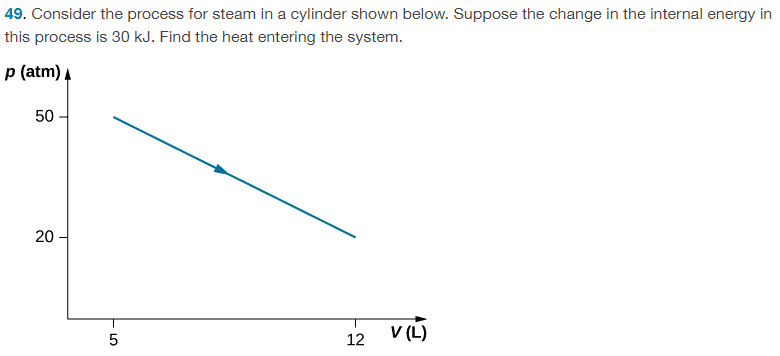# Significant figures -- wrong answer to Thermodynamics question?

• ChiralSuperfields

#### ChiralSuperfields

Homework Statement
I am confused why the answer to the problem below has more significant figures than it should.
Relevant Equations
##\Delta E_{int} = Q - W##
For this problem,The solution is, ## Q = 54500 J ## , however, since the calculation for heat uses the first law which involves ##5 \times 10^{-3) m^3 ## value for the initial volume, should the finial answer not be to 1 sig fig as well? This would give ## 60000 J ##.

Many thanks!

#### Attachments

Whether it is ##5\cdot 10^{-3}##, ##5.0\cdot 10^{-3}##, ##5.00\cdot 10^{-3}## etc. depends on how precisely you think you can read it off the chart. Similarly the other chart readings. The more limiting precision would be the 30kJ.

•ChiralSuperfields
Whether it is ##5\cdot 10^{-3}##, ##5.0\cdot 10^{-3}##, ##5.00\cdot 10^{-3}## etc. depends on how precisely you think you can read it off the chart. Similarly the other chart readings. The more limiting precision would be the 30kJ.
•